# Aeroacoustic Noise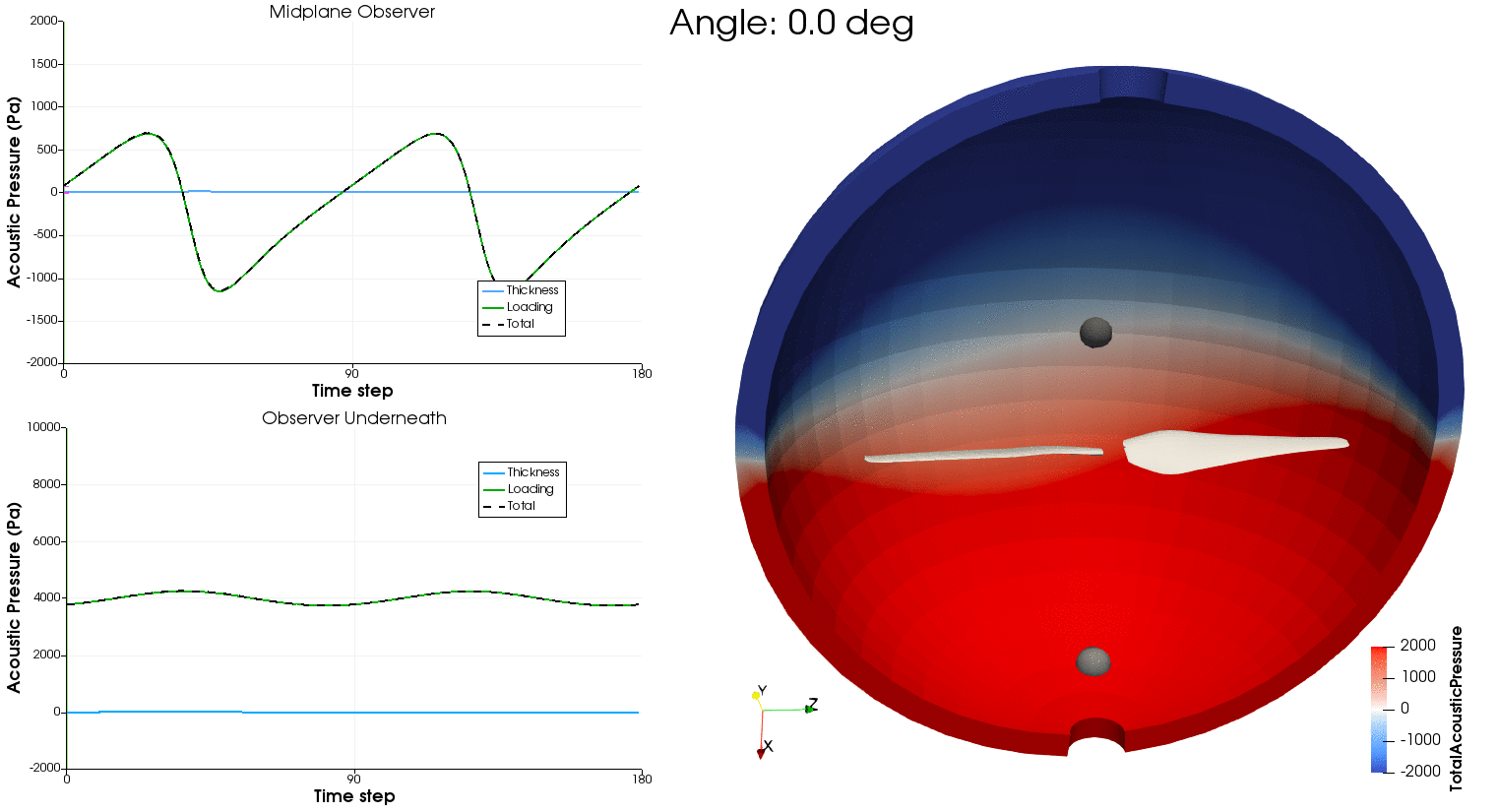Using the aerodynamic solution obtained in the previous section, we can now feed the time-resolved loading and blade motion to PSU-WOPWOP and BPM.jl to compute aeroacoustic noise. PSU-WOPWOP is a Ffowcs Williams-Hawkings acoustic analogy using the time-domain integral Farassat 1A formulation to compute tonal noise from loading and thickness sources (FLOWUnsteady uses a compact representation for the loading source, while using the actual 3D loft of the blade for the thickness source). BPM.jl is an implementation of the semi-empirical methodology developed by Brooks, Pope, and Marcolini to predict broadband noise. The methodology models five self-noise mechanisms due to boundary-layer phenomena: boundary-layer turbulence passing the trailing edge, separated boundary-layer and stalled-airfoil flow, vortex shedding due to laminar-boundary-layer instabilities, vortex shedding from blunt trailing edges, and turbulent flow due to vortex tip formation.

In the following code we exemplify the following:

As a reference, this is the orientation of the rotor and microphone array used in this example: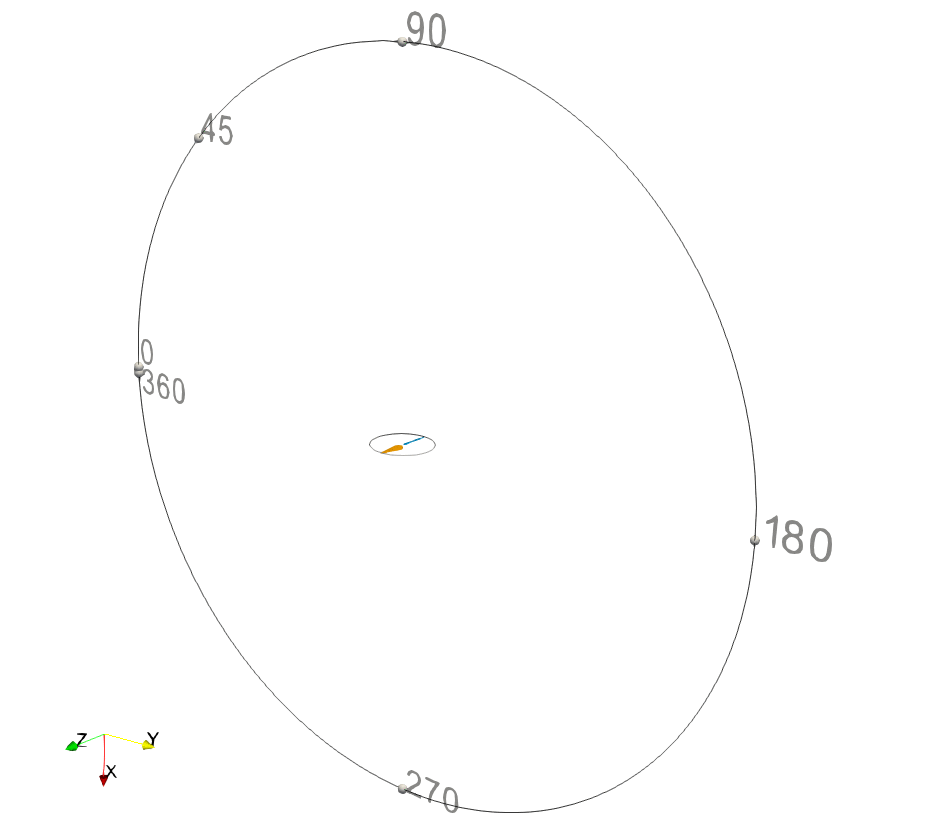PSU-WOPWOP

PSU-WOPWOP is a closed-source code that is not included in FLOWUnsteady, but is graciously made available as a binary by its developers at Penn State University upon inquiry. We recommend contacting them directly to obtain a binary.

FLOWUnsteady has been tested with PSU-WOPWOP v3.4.4.

# Preamble

We load FLOWUnsteady and the FLOWUnsteady.noise module:

import FLOWUnsteady as uns

# Path where to read and save simulation data
sims_path = "/media/edoalvar/T7/simulationdata202304"


# Tonal Noise

These files are read calling uns.run_noise_wopwop, which converts the outputs of the aero solution into a PSU-WOPWOP case. The PSU-WOPWOP binary is then called to read the case and propagate the noise to a set of observers (microphones). The PSU-WOPWOP solution is then written to the same case folder.

# Path from where to read aerodynamic solution
read_path       = joinpath(sims_path, "rotorhover-example-midhigh00") # <-- This must point to you aero simulation

# Path where to save PSU-WOPWOP outputs

# Path to PSU-WOPWOP binary (not included in FLOWUnsteady)
wopwopbin       = "/home/edoalvar/Dropbox/WhisperAero/OtherCodes/PSU-WOPWOP_v3.4.4/wopwop3_linux_serial"

# Run name (prefix of rotor files to read)
run_name        = "singlerotor"

# Make this true if the aero simulation used the quasi-steady solver. If so,
# and will read only one step out of the aero solution
const_solution  = false

# ------------ PARAMETERS ------------------------------------------------------
# NOTE: Make sure that these parameters match what was used in the
#       aerodynamic solution

# Rotor geometry
rotor_file      = "DJI9443.csv"        # Rotor geometry
data_path       = uns.def_data_path    # Path to rotor database

rotorsystems    = [[B]]                # rotorsystems[si][ri] is the number of blades of the ri-th rotor in the si-th system

# Simulation parameters
RPM             = 5400                 # RPM here is a reference value to go from nrevs to simulation time
CW              = false                # Clock-wise rotation of constant geometry (used if const_solution=true)
rho             = 1.071778             # (kg/m^3) air density
speedofsound    = 342.35               # (m/s) speed of sound

# Aero input parameters
nrevs           = 2                    # Number of revolutions to read
nrevs_min       = 6                    # Start reading from this revolution
nsteps_per_rev  = 72                   # Number of steps per revolution in aero solution
num_min         = ceil(Int, nrevs_min*nsteps_per_rev) # Start reading aero files from this step number

if const_solution                       # If constant solution, it overrides to read only the first time step
nrevs       = nothing
nsteps_per_rev = nothing
num_min     = 1
end

# PSU-WOPWOP parameters
ww_nrevs        = 18                     # Number of revolutions in PSU-WOPWOP (18 revs at 5400 RPM gives fbin = 5 Hz)
ww_nsteps_per_rev = max(120, 2*nsteps_per_rev) # Number of steps per revolution in PSU-WOPWOP
const_geometry  = const_solution       # Whether to run PSU-WOPWOP on constant geometry read from num_min
periodic        = true                 # Periodic aerodynamic solution
highpass        = 0.0                  # High pass filter (set this to >0 to get rid of 0th freq in OASPL)

# NOTE: periodic=true assumes that the aero solution is periodic, which allows
#       PSU-WOPWOP to simulate more revolutions (ww_nrevs) that what is
#       read from the aero simulation (nrevs). This is a good assumption for
#       the initial transient state (even with small unsteady fluctuations),
#       but set it to false in simulations with complex control inputs
#       (e.g., maneuvering aircraft, variable RPM, etc). If the outputs of
#       PSU-WOPWOP seem nonsensical, try setting this to false and increasing
#       the number of revolutions in the aero solution (nrevs).

# Observer definition: Circular array of microphones
sph_R           = 1.905                # (m) radial distance from rotor hub
sph_nR          = 0                    # Number of microphones in the radial direction
sph_nphi        = 0                    # Number of microphones in the zenith direction
sph_ntht        = 72                   # Number of microphones in the azimuthal direction
sph_thtmin      = 0                    # (deg) first microphone's angle
sph_thtmax      = 360                  # (deg) last microphone's angle
sph_phimax      = 180
sph_rotation    = [90, 0, 0]           # Rotation of grid of microphones

# NOTE: Here we have defined the microphone array as a circular array, but
#       we could have defined a hemisphere instead by simply making sph_nphi
#       different than zero, or a full volumetric spherical mesh making sph_nR
#       different than zero

# Alternative observer definition: Single microphone
Rmic            = 1.905                # (m) radial distance from rotor hub
anglemic        = 90*pi/180            # (rad) microphone angle from plane of rotation (- below, + above)
# 0deg is at the plane of rotation, 90deg is upstream
microphoneX     = nothing              # Comment and uncomment this to switch from array to single microphone
# microphoneX   = Rmic*[-sin(anglemic), cos(anglemic), 0]

# ------------ RUN PSU-WOPWOP ----------------------------------------------
@time uns.run_noise_wopwop(read_path, run_name, RPM, rho, speedofsound, rotorsystems,
ww_nrevs, ww_nsteps_per_rev, save_ww_path, wopwopbin;
nrevs=nrevs, nsteps_per_rev=nsteps_per_rev,
# ---------- OBSERVERS -------------------------
sph_R=sph_R,
sph_nR=sph_nR, sph_ntht=sph_ntht,
sph_nphi=sph_nphi, sph_phimax=sph_phimax,
sph_rotation=sph_rotation,
sph_thtmin=sph_thtmin, sph_thtmax=sph_thtmax,
microphoneX=microphoneX,
# ---------- SIMULATION OPTIONS ----------------
periodic=periodic,
# ---------- INPUT OPTIONS ---------------------
num_min=num_min,
const_geometry=const_geometry,
axisrot="automatic",
CW=CW,
highpass=highpass,
# ---------- OUTPUT OPTIONS --------------------
verbose=true, v_lvl=0,
prompt=true, debug_paraview=false,
debuglvl=0,                     # PSU-WOPWOP debug level (verbose)
observerf_name="observergrid",  # .xyz file with observer grid
case_name="runcase",            # Name of case to create and run
);

Run time: ~1 minute on a Dell Precision 7760 laptop.

Frequency bins

The length of the frequency bins in the SPL spectrum obtained from the FFT falls out from the following relationships

\begin{align*} f_\mathrm{bin} = \frac{f_\mathrm{sample}}{n_\mathrm{samples}} = \frac{1}{n_\mathrm{samples} \Delta t_\mathrm{sample}} = \frac{\mathrm{RPM}}{60}\frac{1}{n_\mathrm{revs}} .\end{align*}

Thus, in order to obtain the desired frequency bin $f_\mathrm{bin}$, the number of revolutions that PSU-WOPWOP needs to simulate (ww_nrevs) is

\begin{align*} n_\mathrm{revs} = \frac{\mathrm{RPM}}{60}\frac{1}{f_\mathrm{bin}} .\end{align*}

If you need to debug the aero→acoustics workflow, it is useful to convert the input files that we gave to PSU-WOPWOP back to VTK and visualize them in ParaView. This helps verify that we are passing the right things to PSU-WOPWOP. The following lines grab those input files that are formated for PSU-WOPWOP, converts them into VTK files, and opens them in ParaView:


read_ww_path   = joinpath(save_ww_path, "runcase")      # Path to PWW's input files
save_vtk_path  = joinpath(read_ww_path, "vtks")         # Where to save VTK files

# Generate VTK files

println("Generated the following files:\n\t$(vtk_str)") # Call Paraview to visualize VTKs run(paraview --data=$(vtk_str))


Now, we calculate the broadband noise from non-deterministic sources through BPM. This is done calling uns.run_noise_bpm as follows:

# Path where to save BPM outputs
save_bpm_path   = joinpath(sims_path, "rotorhover-example-midhigh00-bpm")

# ------------ PARAMETERS --------------------------------------------------
# NOTE: Make sure that these parameters match what was used in the
#       aerodynamic solution

# Rotor geometry
rotor_file      = "DJI9443.csv"        # Rotor geometry
data_path       = uns.def_data_path    # Path to rotor database
pitch           = 0.0                  # (deg) collective pitch of blades
n               = 50                   # Number of blade elements (this does not need to match the aero solution)
CW              = false                # Clock-wise rotation

# Simulation parameters
RPM             = 5400                 # RPM
J               = 0.0001               # Advance ratio Vinf/(nD)
AOA             = 0                    # (deg) Angle of attack (incidence angle)

rho             = 1.071778             # (kg/m^3) air density
mu              = 1.85508e-5           # (kg/ms) air dynamic viscosity
speedofsound    = 342.35               # (m/s) speed of sound

magVinf         = J*RPM/60*(2*R)
Vinf(X,t)       = magVinf*[cosd(AOA), sind(AOA), 0]  # (m/s) freestream velocity

# BPM parameters
TE_thickness    = 16.0                 # (deg) trailing edge thickness
noise_correction= 1.00                 # Calibration parameter (1 = no correction)
freq_bins       = uns.BPM.default_f    # Frequency bins (default is one-third octave band)

# Observer definition: Circular array of microphones
sph_R           = 1.905                # (m) radial distance from rotor hub
sph_nR          = 0                    # Number of microphones in the radial direction
sph_nphi        = 0                    # Number of microphones in the zenith direction
sph_thtmin      = 0                    # (deg) first microphone's angle
sph_thtmax      = 360                  # (deg) last microphone's angle
sph_phimax      = 180
sph_rotation    = [90, 0, 0]           # Rotation of grid of microphones

# Alternative observer definition: Single microphone
Rmic            = 1.905                # (m) radial distance from rotor hub
anglemic        = 90*pi/180            # (rad) microphone angle from plane of rotation (- below, + above)
# 0deg is at the plane of rotation, 90deg is upstream
microphoneX     = nothing              # Comment and uncomment this to switch from array to single microphone
# microphoneX   = Rmic*[-sin(anglemic), cos(anglemic), 0]

# ------------ GENERATE GEOMETRY -------------------------------------------
# Generate rotor
rotor = uns.generate_rotor(rotor_file; pitch=pitch,
n=n, CW=CW, ReD=0.0,
verbose=false, xfoil=false,
data_path=data_path,
plot_disc=false)

rotors = vlm.Rotor[rotor]              # All rotors in the computational domain

# ------------ RUN BPM -----------------------------------------------------
uns.run_noise_bpm(rotors, RPM, Vinf, rho, mu, speedofsound,
save_bpm_path;
# ---------- OBSERVERS -------------------------
sph_R=sph_R,
sph_nR=sph_nR, sph_ntht=sph_ntht,
sph_nphi=sph_nphi, sph_phimax=sph_phimax,
sph_rotation=sph_rotation,
sph_thtmin=sph_thtmin, sph_thtmax=sph_thtmax,
microphoneX=microphoneX,
# ---------- BPM OPTIONS -----------------------
noise_correction=noise_correction,
TE_thickness=TE_thickness,
freq_bins=freq_bins,
# ---------- OUTPUT OPTIONS --------------------
prompt=true
);


# Results

Finally, we add tonal and broadband noise together and plot the results

We start by reading the outputs from PSU-WOPWOP and BPM:

# Dataset to read and associated information
dataset_infos = [ # (label, PWW solution, BPM solution, line style, color)
joinpath(sims_path, "rotorhover-example-midhigh00-pww/runcase/"),
joinpath(sims_path, "rotorhover-example-midhigh00-bpm"),
"-", "steelblue"),
]

datasets_pww = Dict()     # Stores PWW data in this dictionary
datasets_bpm = Dict()     # Stores BPM data in this dictionary

# Read datasets and stores them in dictionaries

println("Done!")


Also, we need to recreate the circular array of microphones that was used when generating the aeroacoustic solutions:

# These parameters will be used for plotting
RPM          = 5400                  # RPM of solution

# Make sure this grid is the same used as an observer by the aeroacoustic solution
sph_R        = 1.905                 # (m) radial distance from rotor hub
sph_nR       = 0
sph_nphi     = 0
sph_ntht     = 72                    # Number of microphones
sph_thtmin   = 0                     # (deg) first microphone's angle
sph_thtmax   = 360                   # (deg) last microphone's angle
sph_phimax   = 180
sph_rotation = [90, 0, 0]            # Rotation of grid of microphones

# Create observer grid
grid = noise.observer_sphere(sph_R, sph_nR, sph_ntht, sph_nphi;
thtmin=sph_thtmin, thtmax=sph_thtmax, phimax=sph_phimax,
rotation=sph_rotation);

# This function calculates the angle that corresponds to every microphone
pangle(i) = -180/pi*atan(gt.get_node(grid, i), gt.get_node(grid, i))


### Pressure waveform

Here we plot the pressure waveform at some of the microphones. This pressure waveform includes only the tonal component, as given by PSU-WOPWOP.

microphones  = [-45, 90]            # (deg) microphones to plot

noise.plot_pressure(dataset_infos, microphones, RPM, sph_ntht, pangle;
datasets_pww=datasets_pww, xlims=[0, 5])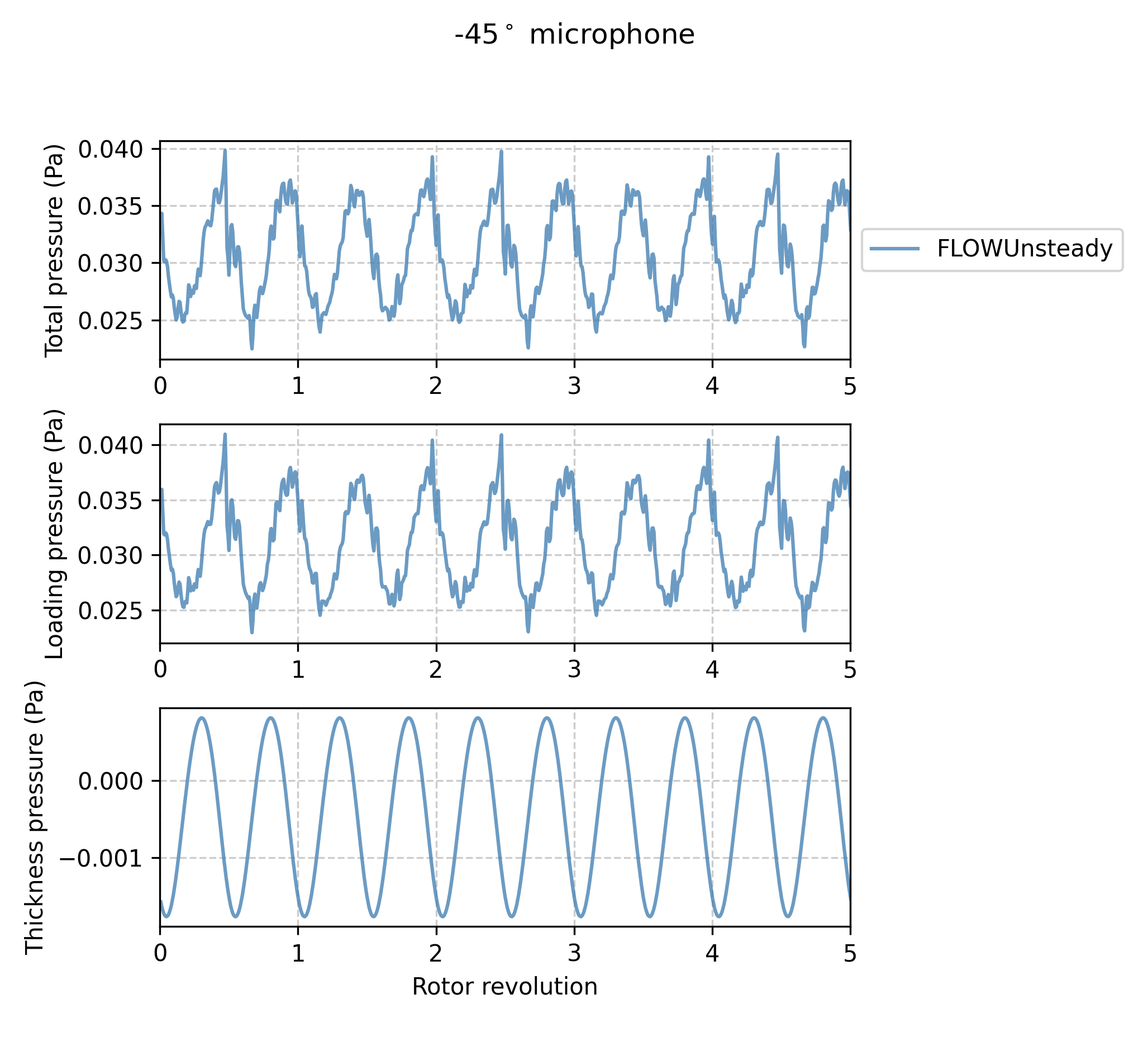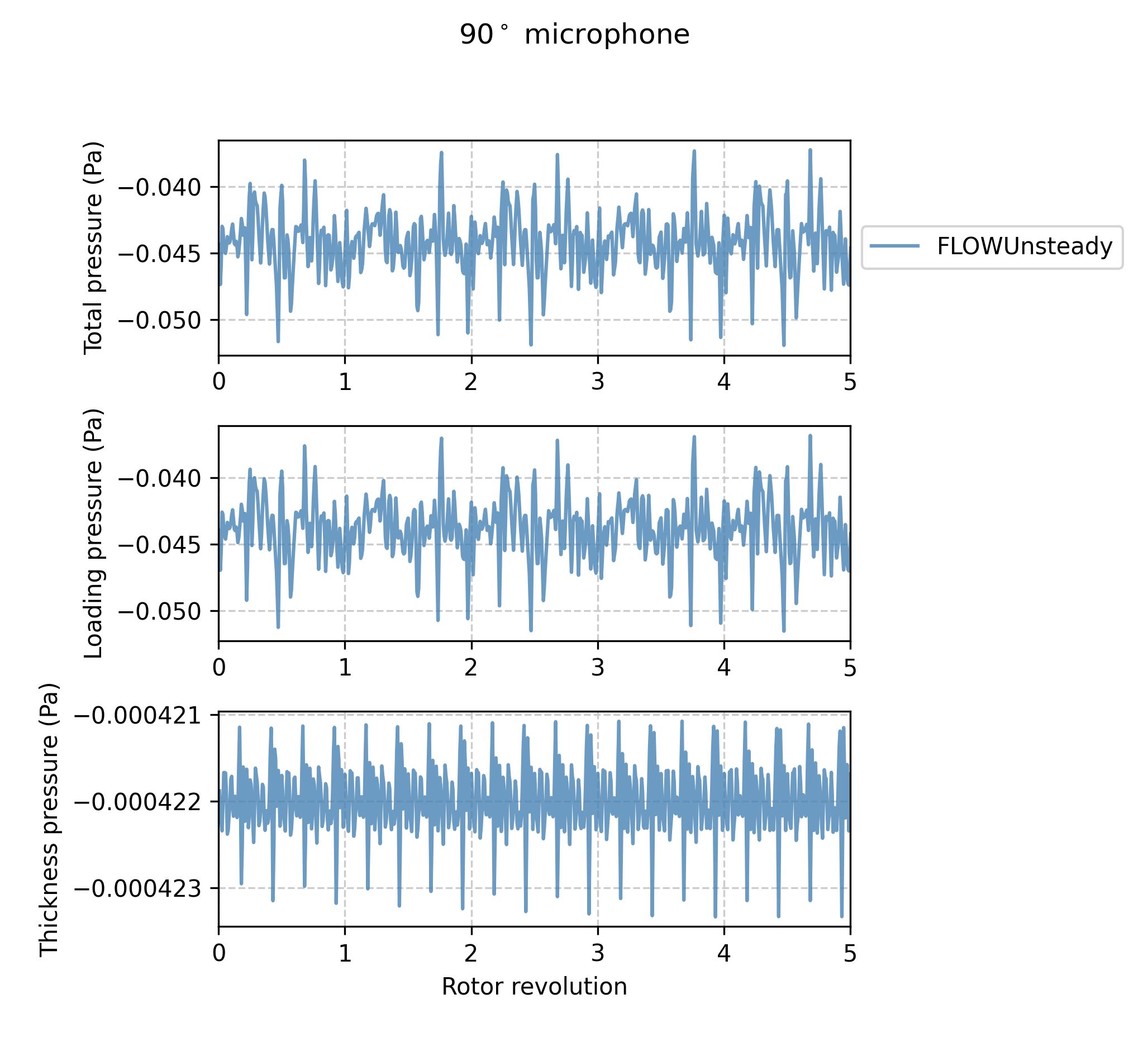### SPL Spectrum

We now compare the (sound pressure level) SPL spectrum at the microphone $-45^\circ$ below the plane of rotation with the experimental data reported by Zawodny et al..

One-third octave band:

microphones  = [-45]                # (deg) microphone to plot
Aweighted    = false                # Plot A-weighted SPL
onethirdoctave = true               # Plot 1/3 octave band

# Experimental data from Zawodny et al., Fig. 9
zawodny_path = joinpath(uns.examples_path, "..", "docs", "resources", "data", "zawodny2016")
exp_filename = joinpath(zawodny_path, "zawodny_dji9443-fig9-OTO-5400.csv")
plot_experimental = [(exp_filename, "Experimental", "o:k", Aweighted, [])]

# Plot SPL spectrum
noise.plot_spectrum_spl(dataset_infos, microphones, BPF, sph_ntht, pangle;
datasets_pww=datasets_pww, datasets_bpm=datasets_bpm,
Aweighted=Aweighted,
onethirdoctave=onethirdoctave,
plot_csv=plot_experimental,
xBPF=false, xlims=[100, 3e4], ylims=[0, 80], BPF_lines=8)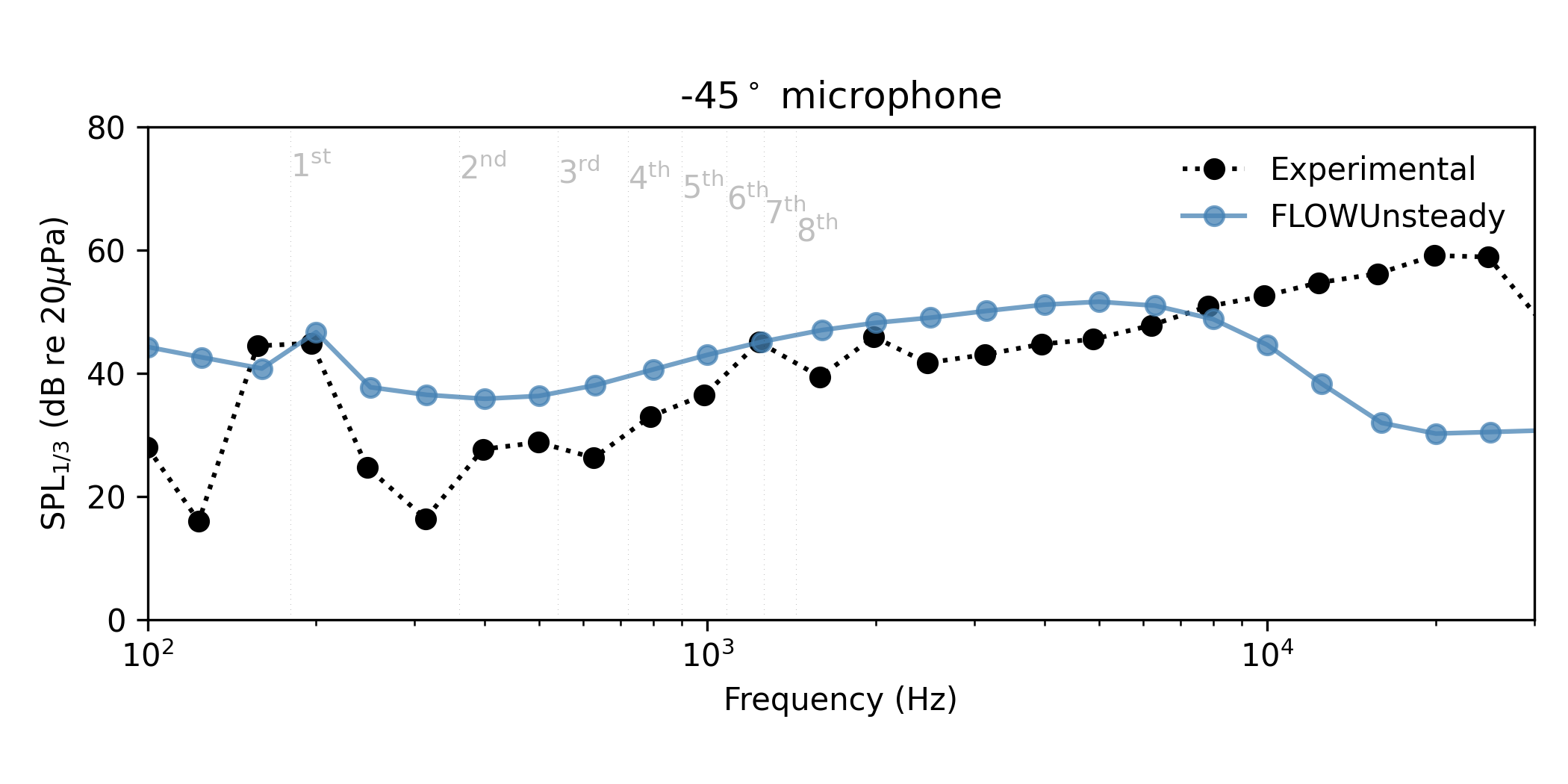A-weighted narrow band:

Aweighted    = true                # Plot A-weighted SPL

# Experimental data from Zawodny et al., Fig. 9
exp_filename = joinpath(zawodny_path, "zawodny_dji9443_spl_5400_01.csv")
plot_experimental = [(exp_filename, "Experimental", "k", Aweighted, [])]

# Plot SPL spectrum
noise.plot_spectrum_spl(dataset_infos, microphones, BPF, sph_ntht, pangle;
datasets_pww=datasets_pww, datasets_bpm=datasets_bpm,
Aweighted=Aweighted,
plot_csv=plot_experimental,
xBPF=false, xlims=[100, 3e4], BPF_lines=21)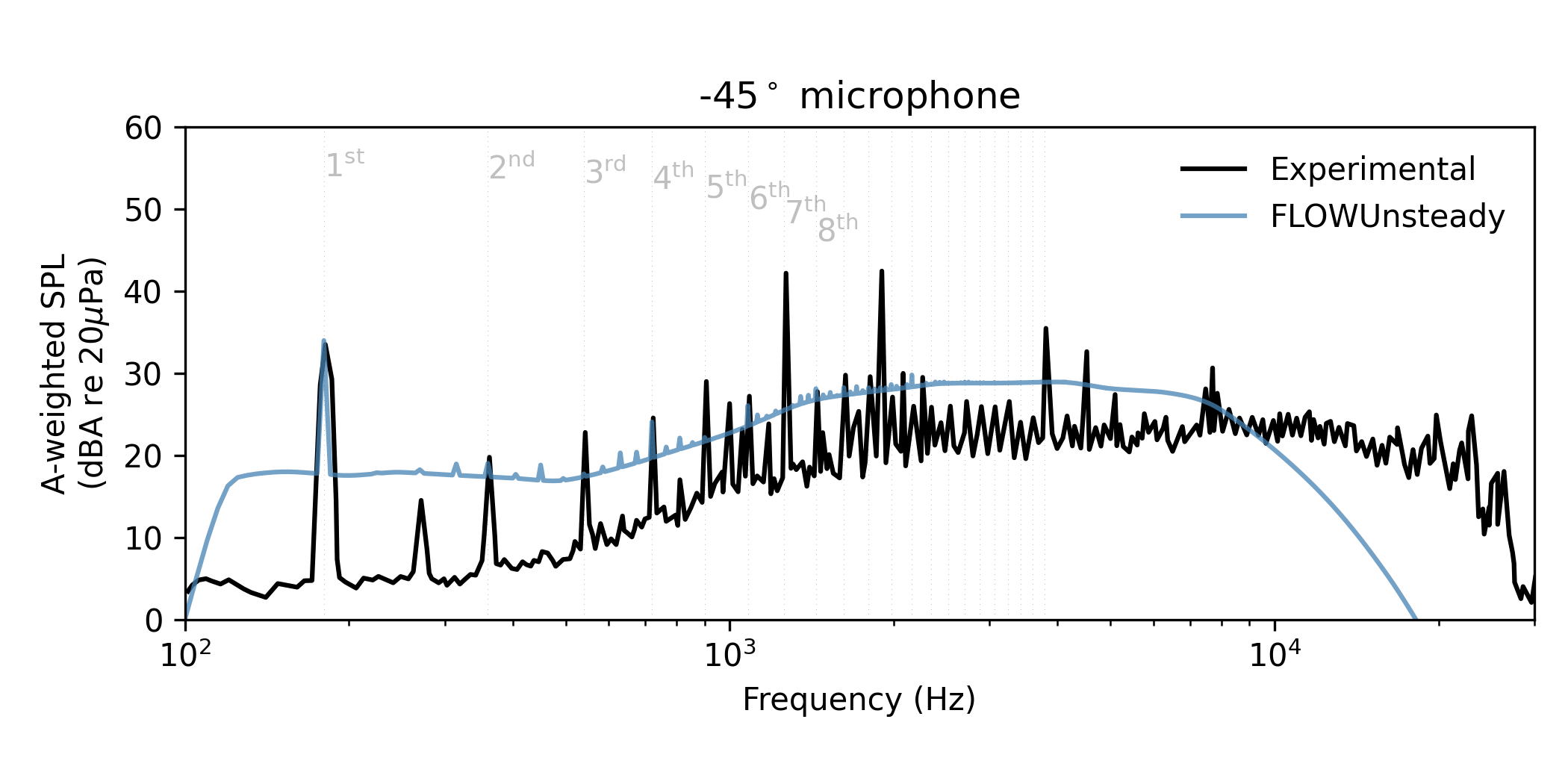In the narrow band spectrum, keep in mind that the broadband output of BPM has been converted from 1/3 octaves into narrow band (5 hz bin) in order to plot it together with the tonal noise, but this is a very rough approximation (we lack enough information to interpolate from a coarse band to a narrow band besides just smearing the energy content). Hence, the broadband component seems to overpredict relative to the experimental, but this is an artifice of the 1/3 octave $\rightarrow$ narrow band conversion. In the OASPL directivity plots we will see that the A-weighted OASPL predicted with BPM actually matches the experimental values very well.

### Tonal noise

Here we plot only the tonal component of noise associated with harmonics of the blade passing frequency (BPF),

Tonal SPL spectrum

Aweighted       = false

# Experimental data from Zawodny et al., Fig. 9
exp_filename = joinpath(zawodny_path, "zawodny_dji9443_spl_5400_01.csv")
plot_experimental = [(exp_filename, "Experimental", "k", Aweighted, [])]

# Plot SPL spectrum
noise.plot_spectrum_spl(dataset_infos, microphones, BPF, sph_ntht, pangle;
datasets_pww=datasets_pww, datasets_bpm=datasets_bpm,
Aweighted=Aweighted,
plot_csv=plot_experimental,
xBPF=false, xlims=[100, 3e4], BPF_lines=21)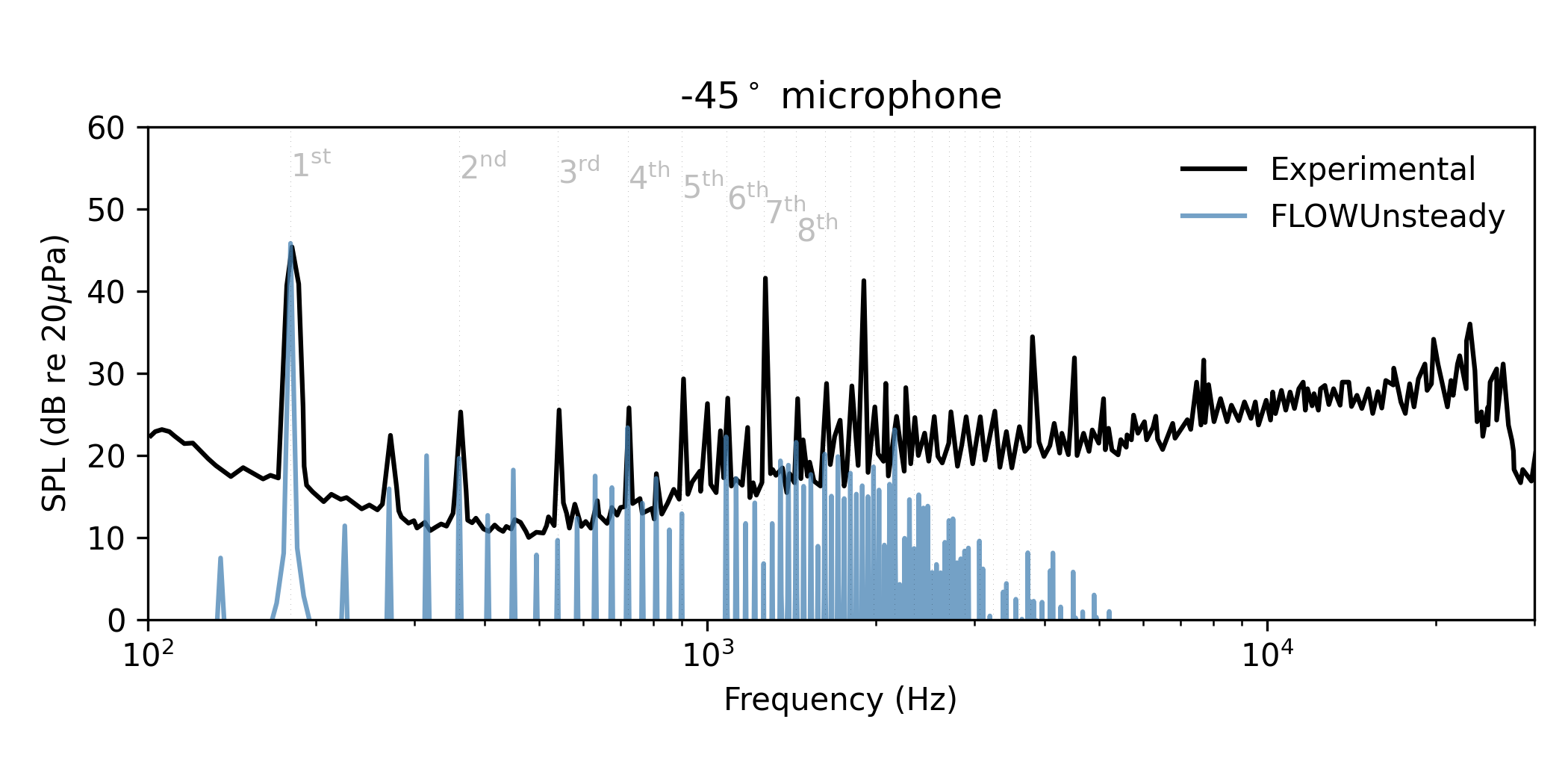Directivity of $1^\mathrm{st}$ BPF

BPFi = 1                 # Multiple of blade-passing frequency to plot

# Experimental and computational data reported by Zawodny et al., Fig. 14
filename1 = joinpath(zawodny_path, "zawodny_fig14_topright_exp00.csv")
filename2 = joinpath(zawodny_path, "zawodny_fig14_topright_of00.csv")
filename3 = joinpath(zawodny_path, "zawodny_fig14_topright_pas00.csv")

plot_experimental = [(filename1, "Experimental", "ok", Aweighted, []),
(filename2, "OVERFLOW2", "-y", Aweighted, [(:alpha, 0.8)])]

# Plot SPL directivity of first blade-passing frequency
noise.plot_directivity_splbpf(dataset_infos, BPFi, BPF, pangle;
datasets_pww=datasets_pww,
datasets_bpm=datasets_bpm,
plot_csv=plot_experimental,
rticks=40:4:52, rlims=[40, 54], rorigin=36)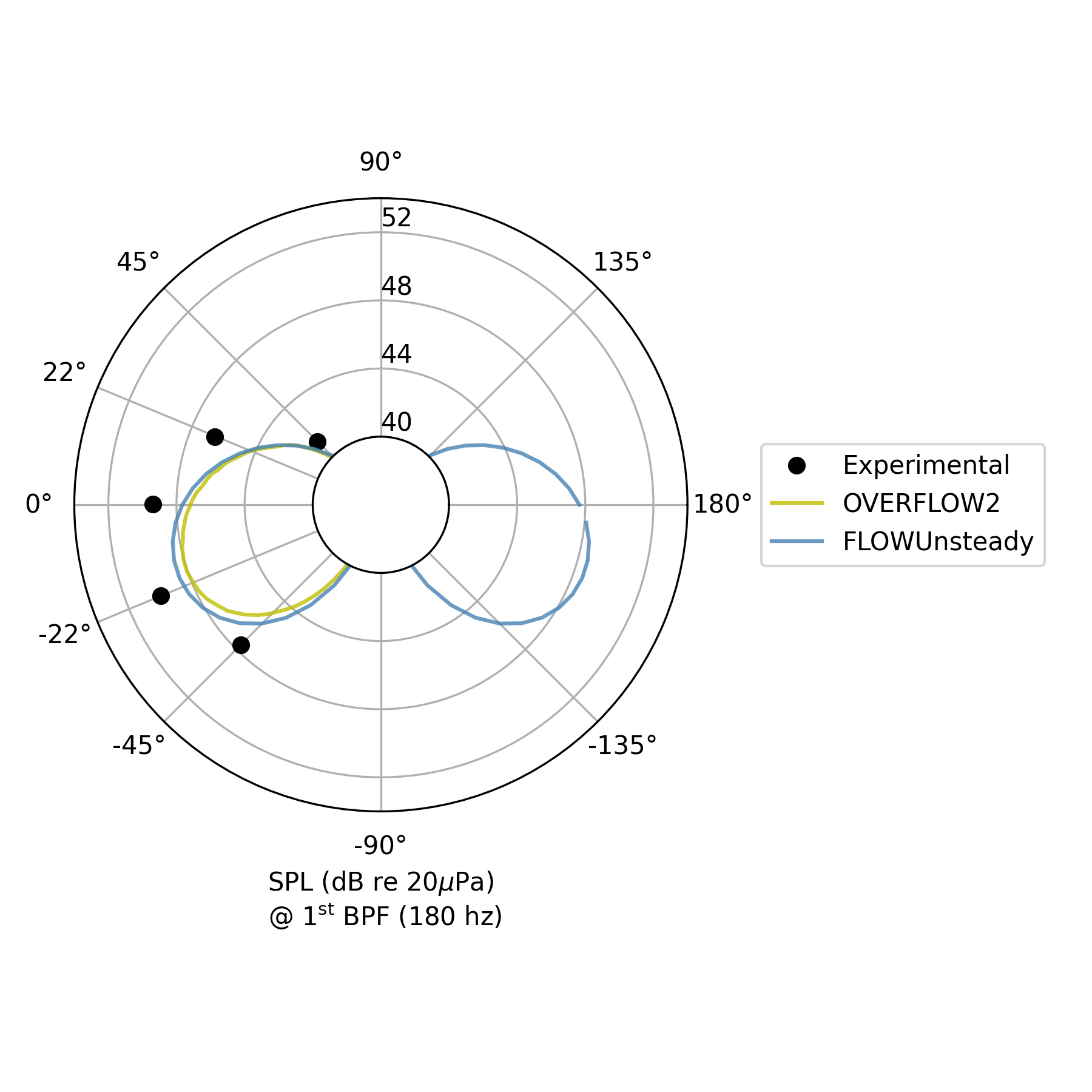Directivity of $2^\mathrm{nd}$ BPF

BPFi = 2                 # Multiple of blade-passing frequency to plot

# Experimental and computational data reported by Zawodny et al., Fig. 14
filename1 = joinpath(zawodny_path, "zawodny_fig14_bottomright_exp00.csv")
filename2 = joinpath(zawodny_path, "zawodny_fig14_bottomright_of00.csv")
filename3 = joinpath(zawodny_path, "zawodny_fig14_bottomright_pas00.csv")

plot_experimental = [(filename1, "Experimental", "ok", Aweighted, []),
(filename2, "OVERFLOW2", "-y", Aweighted, [(:alpha, 0.8)])]

# Plot SPL directivity of first blade-passing frequency
noise.plot_directivity_splbpf(dataset_infos, BPFi, BPF, pangle;
datasets_pww=datasets_pww,
datasets_bpm=datasets_bpm,
plot_csv=plot_experimental,
rticks=15:5:30, rlims=[0, 32], rorigin=0)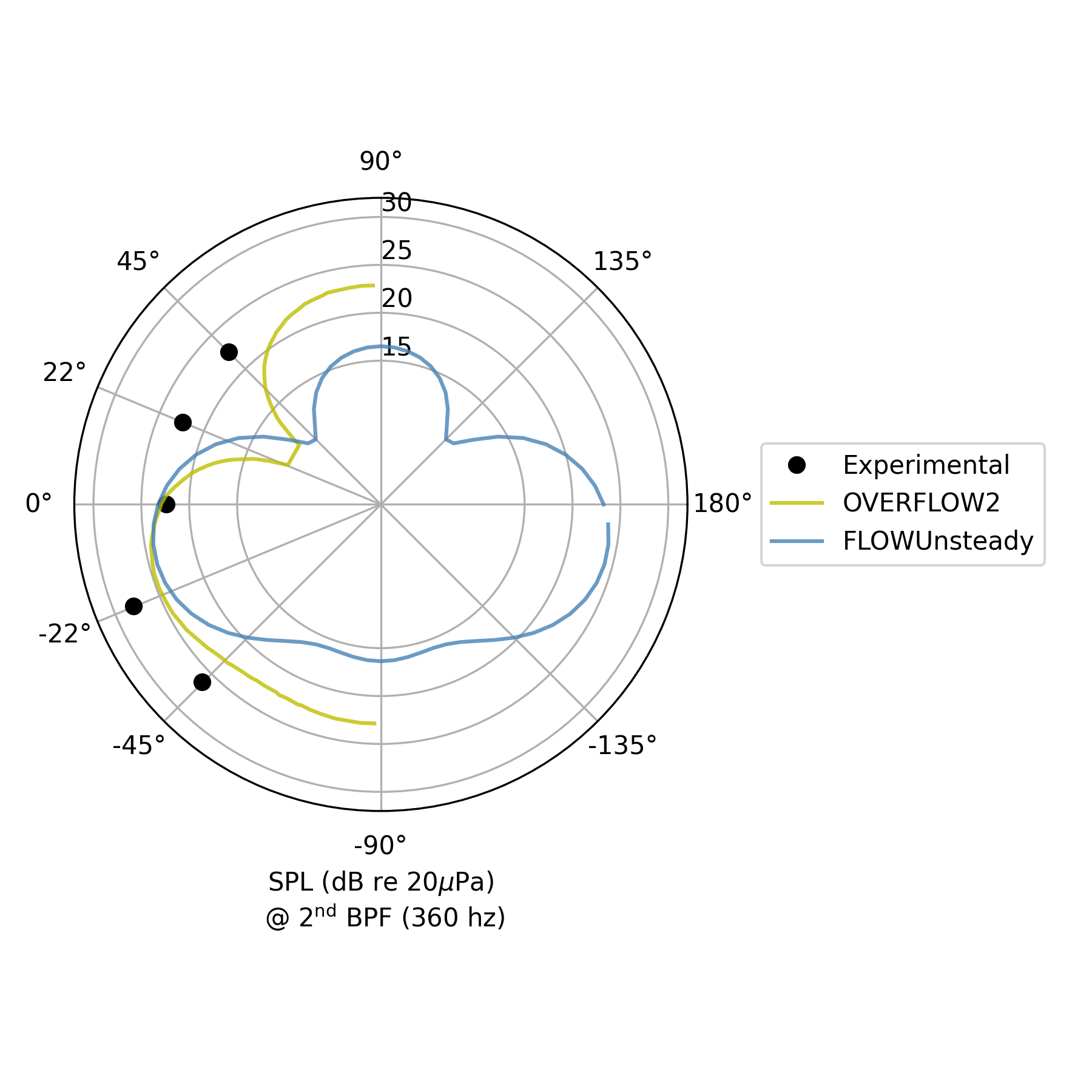### OASPL directivity

Unweighted overall SPL (OASPL)

Aweighted = false

# Experimental and computational data reported by Zawodny et al., Fig. 12
exp_filename = joinpath(zawodny_path, "zawodny_fig12_left_5400_00.csv")
plot_experimental = [(exp_filename, "Experimental", "ok", Aweighted, [])]

# Plot OASPL directivity
noise.plot_directivity_oaspl(dataset_infos, pangle;
datasets_pww=datasets_pww,
datasets_bpm=datasets_bpm,
Aweighted=Aweighted,
plot_csv=plot_experimental,
rticks=40:10:70, rlims=[40, 72], rorigin=30)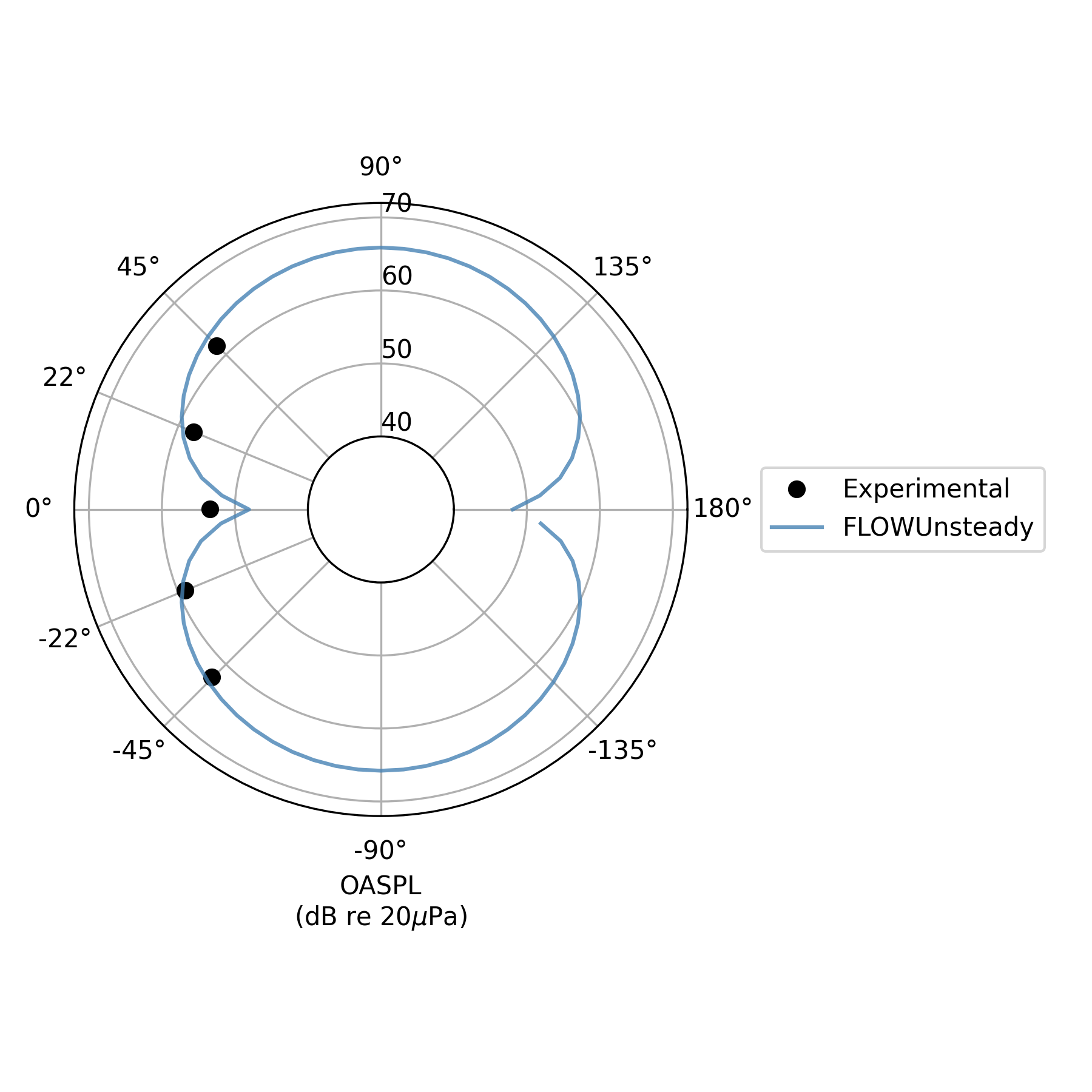A-weighted OASPL

Aweighted = true

# Experimental and computational data reported by Zawodny et al., Fig. 12
exp_filename = joinpath(zawodny_path, "zawodny_fig12_right_5400_00.csv")
plot_experimental = [(exp_filename, "Experimental", "ok", Aweighted, [])]

# Plot OASPL directivity
noise.plot_directivity_oaspl(dataset_infos, pangle;
datasets_pww=datasets_pww,
datasets_bpm=datasets_bpm,
Aweighted=Aweighted,
plot_csv=plot_experimental,
rticks=40:10:70, rlims=[40, 72], rorigin=30)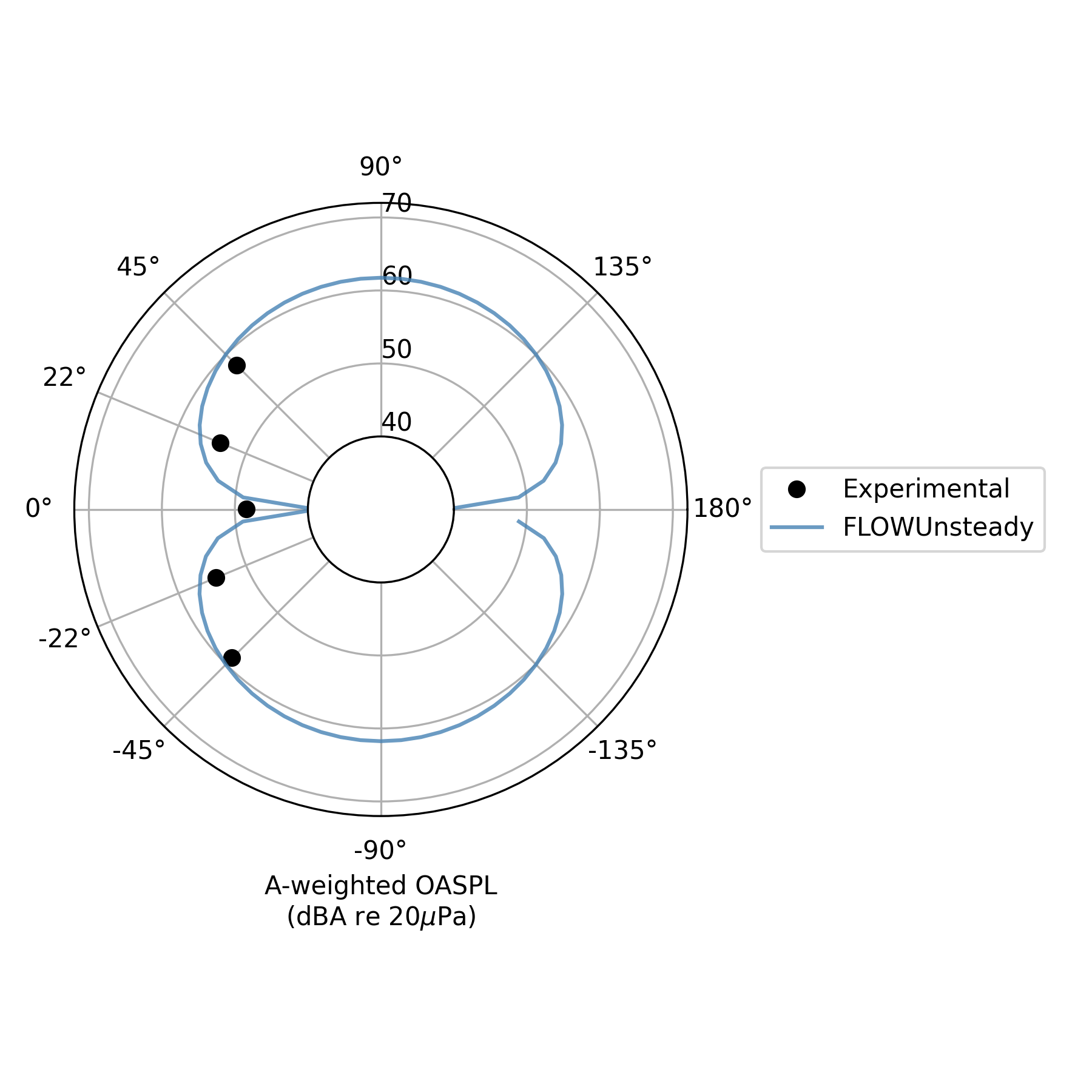• 1N. S. Zawodny, D. D. Boyd, Jr., and C. L. Burley, “Acoustic Characterization and Prediction of Representative, Small-scale Rotary-wing Unmanned Aircraft System Components,” in 72nd American Helicopter Society (AHS) Annual Forum (2016).